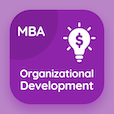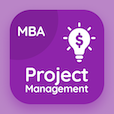The Book Forecast Accuracy Multiple Choice Questions (MCQ Quiz) with answers, Forecast Accuracy MCQ Quiz PDF download to study online statistics degree courses. Study Time Series Analysis and Forecasting Multiple Choice Questions and Answers (MCQs), Forecast Accuracy quiz answers PDF for part time MBA programs. The e-Book Forecast Accuracy MCQ App Download: moving averages and exponential smoothing, time series patterns test prep for top ranked MBA programs.

The MCQ: A measure of forecast accuracy that avoids the problem of positive/negative forecast errors offsetting one another is known as PDF, "Forecast Accuracy" App Download (Free) with mean standard error, mean absolute error, mean residual factor, and mean estimated factor choices for part time MBA programs. Practice forecast accuracy quiz questions, download Google eBook (Free Sample) .

## Statistics MCQs: Forecast Accuracy Quiz Questions

MCQ: A measure of forecast accuracy that avoids the problem of positive/negative forecast errors offsetting one another is known as

A) Mean standard error
B) Mean absolute error
C) Mean residual factor
D) Mean estimated factor

MCQ: How well a particular forecasting method is able to reproduce the available time series data can be determined by

A) Pattern plot
B) Forecast accuracy
C) Prediction
D) Error factor

MCQ: Forecast error in finding forecast accuracy can be computed as

A) Forecast value- Actual value
B) Actual value- error value
C) Error value- Actual value
D) Actual value-forecast

MCQ: The key concept associated with measuring forecast accuracy is known to be

A) Future value prediction
B) Actual Value
C) Forecast error
D) Residual forecast

MCQ: A simple measure of forecast accuracy is named as

A) Mean of forecast errors
B) Mean sum of squares
C) Mean residual of forecast
D) Mean of forecast deviation

### Practice Tests: MBA Business Statistics Exam Prep

Download MBA Business Statistics Quiz App, Organizational Structure and Design MCQ App, and Project Management MCQs App to install for Android & iOS devices. These Apps include complete analytics of real time attempts with interactive assessments. Download Play Store & App Store Apps & Enjoy 100% functionality with subscriptions!